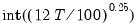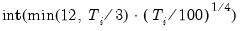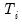uroot
Carries out unit root tests on a series or panel structured series.
For ordinary series, computes conventional Augmented Dickey-Fuller (ADF), GLS detrended Dickey-Fuller (DFGLS), Phillips-Perron (PP), Kwiatkowski, et. al. (KPSS), Elliot, Rothenberg, and Stock (ERS) Point Optimal, or Ng and Perron (NP) tests for a unit root in the series or its first or second difference.
For series in a panel structured workfile, computes Levin, Lin and Chu (LLC), Breitung, Im, Pesaran, and Shin (IPS), Fisher - ADF, Fisher - PP, or Hadri panel unit root tests on levels, first, or second differences of the data.
Syntax
series_name.uroot(options)
There are different options for conventional tests on an ordinary series and panel tests for series in panel structured workfiles.
Options for Conventional Unit Root Tests
Basic Specification
You should specify the exogenous variables and order of dependent variable differencing in the test equation using the following options:

 exog=arg (default=“const”) Specification of exogenous trend variables in the test equation: “const” “trend” (include a constant and a linear time trend), “none” (do not include any exogenous regressors). dif=integer (default=0) Order of differencing of the series prior to running the test. Valid values are {0, 1, 2}.
You should specify the test type using one of the following keywords:

 adf (default) Augmented Dickey-Fuller. dfgls GLS detrended Dickey-Fuller (Elliot, Rothenberg, and Stock). pp Phillips-Perron. kpss Kwiatkowski, Phillips, Schmidt, and Shin. ers Elliot, Rothenberg, and Stock (Point Optimal). np Ng and Perron.
Note that for backward compatibility, EViews supports older forms of the exogenous specification:

 const, c (default) Include a constant in the test equation. trend, t Include a constant and a linear time trend in the test equation. none, n Do not include a constant or time trend (only available for the ADF and PP tests).
For future compatibility we recommend that you use the “exog=” format.
Spectral Estimation Option
In addition, PP, KPSS, ERS, and NP tests all require the estimation of the long-run variance (frequency zero spectrum). You may specify the method using the “hac=” option. The default setting depends on the selected test.

 hac=arg (default=varies) Method of estimating the frequency zero spectrum: “bt” (Bartlett kernel), “pr” (Parzen kernel), “qs” (Quadratic Spectral kernel), “ar” (AR spectral), “ardt (AR spectral - OLS detrended data), “argls” (AR spectral - GLS detrended data).The default settings are test specific (“bt” for PP and KPSS tests, “ar” for ERS, “argls” for NP).
Lag Difference Options
Applicable to ADF and DFGLS tests, and for PP, KPSS, ERS, and NP tests that use a AR spectral density estimator (“hac=ar”, “hac=ardt”, or “hac=argls”). The default lag selection method is based on a comparison of Schwarz criterion values. You may specify a fixed lag using the “lag=” option.

 lagmethod=arg (default=“sic”) Method for selecting lag length (number of first difference terms) to be included in the Dickey-Fuller test regression or number of lags in the AR spectral density estimator:“aic” (Akaike), “sic” (Schwarz), “hqc” (Hannan-Quinn), “msaic” (Modified Akaike), “msic” (Modified Schwarz), “mhqc” (Modified Hannan-Quinn), “tstat” (Ng-Perron first backward significant t-statistic). lag=integer Use-specified fixed lag. maxlag=integer Maximum lag length to consider when performing automatic lag length selection.default=lagpval=arg (default=0.1) Probability value for use in the t-statistic automatic lag selection method (“lagmethod = tstat”).
Kernel Option
Applicable to PP, KPSS, ERS, and NP tests when using kernel estimators of the frequency zero spectrum (where “hac=bt”, “hac=pz”, or “hac=qs”)

 band = arg, b=arg (default=“nw”) Method of selecting the bandwidth: “nw” (Newey-West automatic variable bandwidth selection), “a” (Andrews automatic selection), number (user specified bandwidth).
General Options

 prompt Force the dialog to appear from within a program. p Print output from the test.
Options for Panel Unit Root Tests
Basic Specification
You should specify the exogenous variables, order of dependent variable differencing, and sample handling, in the test equation using the following options:

 exog=arg (default=“const”) Specification of exogenous trend variables in the test equation: “const” (constant),“trend” (include a constant and a linear time trend), “none” (do not include exogenous regressors). dif=integer (default=0) Order of differencing of the series prior to running the test. Valid values are {0, 1, 2}. balance Use balanced (across cross-sections or series) data when performing test.
You may use one of the following keywords to specify the test:

 sum (default) Summary of all of the panel unit root tests. llc Levin, Lin, and Chu. breit Breitung. ips Im, Pesaran, and Shin. adf Fisher - ADF. pp Fisher - PP. hadri Hadri.
For backward compatibility, EViews supports older forms of the exogenous specification:

 const, c (default) Include a constant in the test equation. trend, t Include a constant and a linear time trend in the test equation. none, n Do not include a constant or time trend (only available for the ADF and PP tests).
For future compatibility we recommend that you use the “exog=” format.
Lag Difference Options
Specifies the number of lag difference terms to be included in the test equation. Applicable in “Summary”, LLC, Breitung, IPS, and Fisher-ADF tests. The default setting is to perform automatic lag selection using the Schwarz criteria (“lagmethod=sic”).

 lagmethod=arg (default=“sic”) Method for selecting lag lengths (number of first difference terms) to be included in the Dickey-Fuller test regressions:“aic” (Akaike), “sic” (Schwarz), “hqc” (Hannan-Quinn), “tstat” (Ng-Perron first backward significant t-statistic). lag=arg Specified lag length (number of first difference terms) to be included in the regression: integer (user-specified common lag length), vector_name (user-specific individual lag length, one row per cross-section). maxlag=arg Maximum lag length to consider when performing automatic lag length selection: integer (common maximum lag length), or vector_name (individual maximum lag length, one row per cross-section). The default setting produces individual maximum lags of,default=whereis the length of the cross-section. lagpval=arg (default=0.1) Probability value for use in the t-statistic automatic lag selection method (when “lagmethod = tstat”).
Kernel Options
Specifies options for computing kernel estimates of the zero-frequency spectrum (long-run covariance). Applicable to “Summary”, LLC, Fisher-PP, and Hadri tests.

 hac=arg (default=“bt”) Method of estimating the frequency zero spectrum: “bt” (Bartlett kernel), “pr” (Parzen kernel), “qs” (Quadratic Spectral kernel), band = arg, b=arg (default=“nw”) Method of selecting the bandwidth: “nw” (Newey-West automatic variable bandwidth selection), “a” (Andrews automatic selection), number (user-specified common bandwidth), vector_name (user-specified individual bandwidths, one row for each cross-section).
General options

 prompt Force the dialog to appear from within a program. p Print output from the test.
Examples
The command: